This is a worked set of answers to the ggplot course

# Exercise 1 - Simple point and line plots

First we are going to load the main tidyverse library.

``library(tidyverse)``
``## -- Attaching packages ---------------------------------------------------------------------------------------------- tidyverse 1.3.0 --``
``````## v ggplot2 3.2.1     v purrr   0.3.3
## v tibble  2.1.3     v dplyr   0.8.3
## v tidyr   1.0.2     v stringr 1.4.0
## v readr   1.3.1     v forcats 0.4.0``````
``````## -- Conflicts ------------------------------------------------------------------------------------------------- tidyverse_conflicts() --

## Weight chart

We’ll plot out the data in the `weight_chart.txt` file. Let’s load it and look first.

``read_tsv("weight_chart.txt") -> weight``
``````## Parsed with column specification:
## cols(
##   Age = col_double(),
##   Weight = col_double()
## )``````
``weight``

``````weight %>%
ggplot(aes(x=Age, y=Weight)) +
geom_point()``````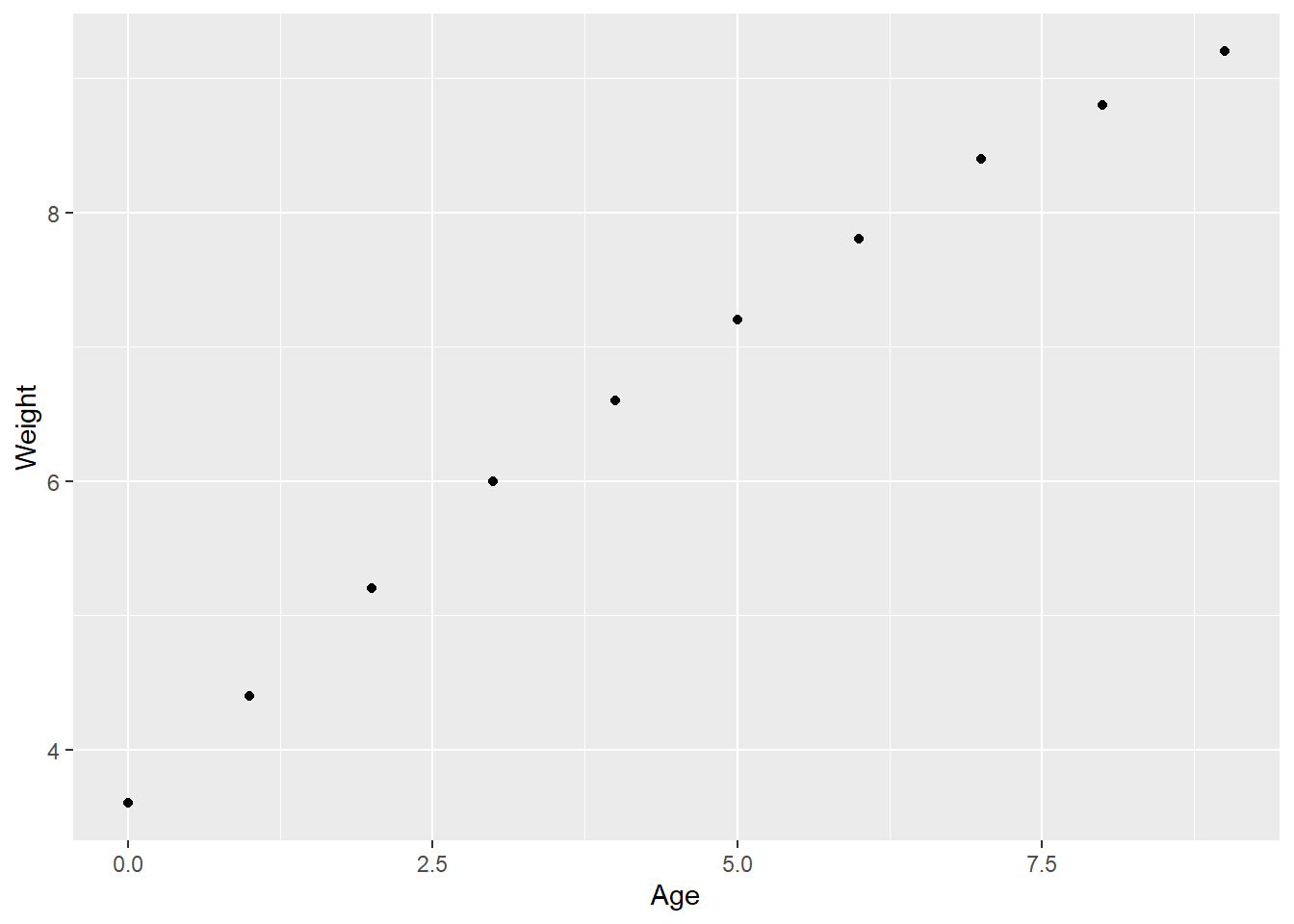Now we can customise this a bit by adding fixed aesthetics to the `geom_point()` function.

``````weight %>%
ggplot(aes(x=Age, y=Weight)) +
geom_point(size=3, colour="blue2")``````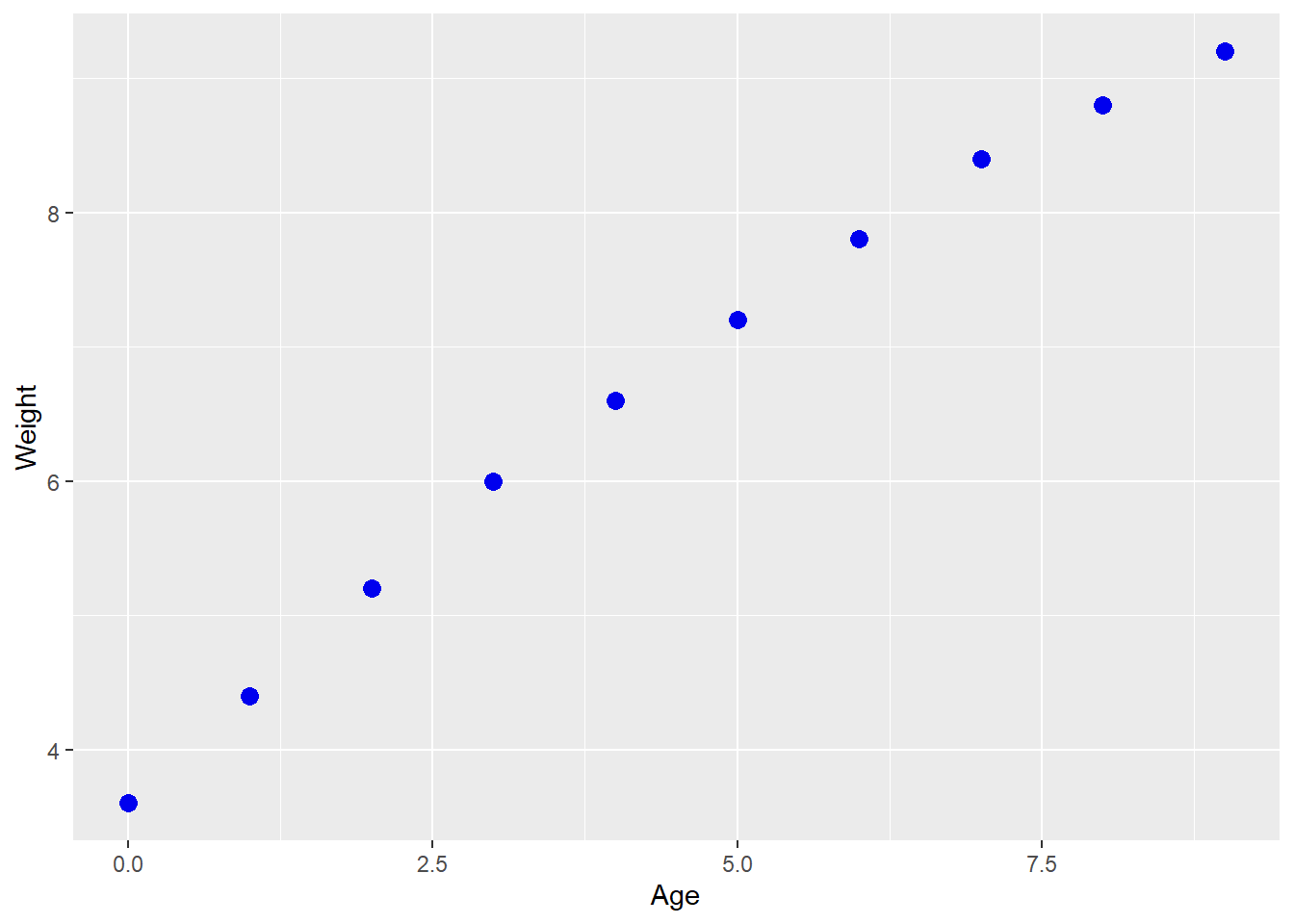Now repeat but with a different geometry.

``````weight %>%
ggplot(aes(x=Age, y=Weight)) +
geom_line()``````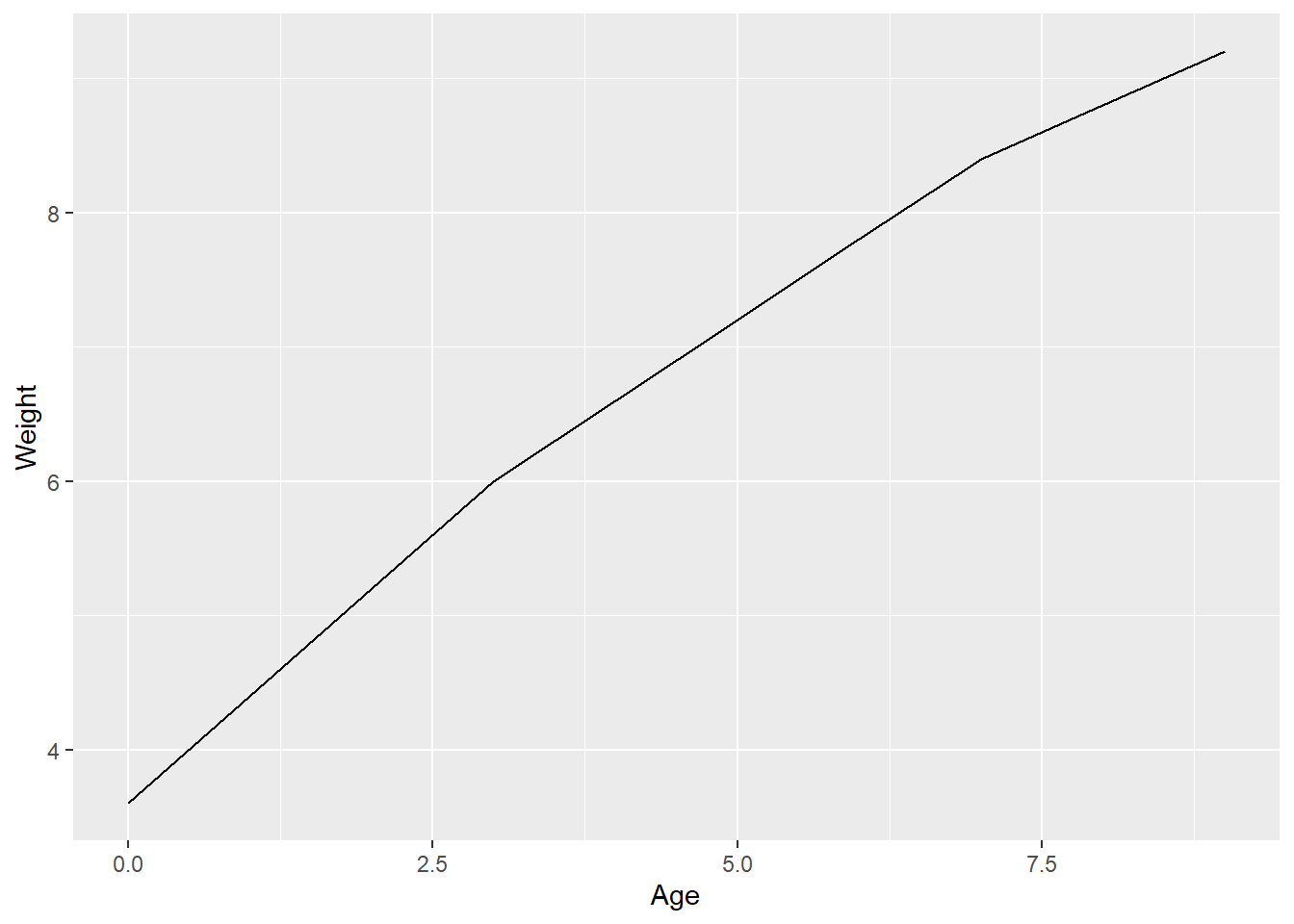Finally, combine the two geometries.

``````weight %>%
ggplot(aes(x=Age, y=Weight)) +
geom_line()+
geom_point(size=3, colour="blue2")``````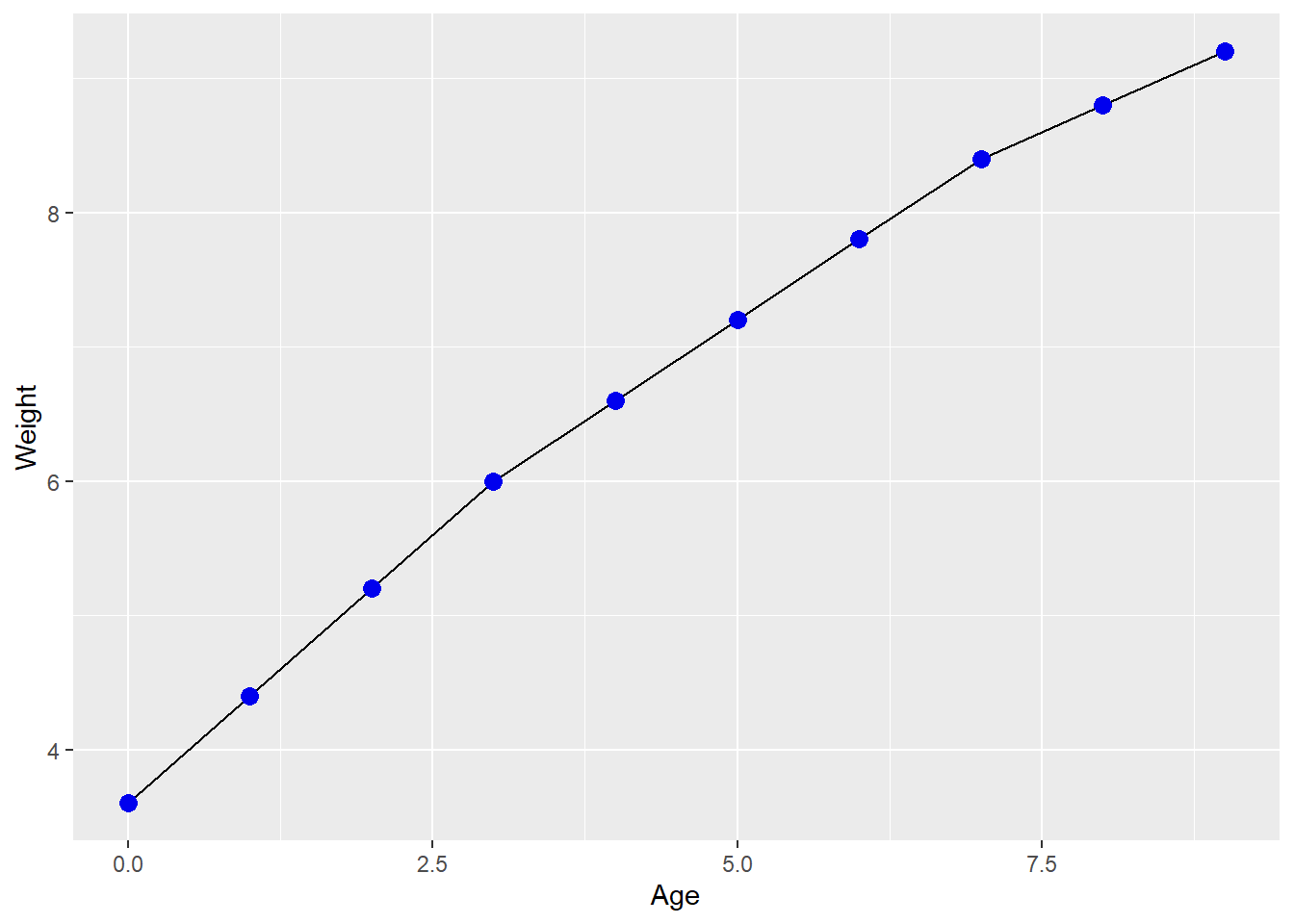## Chromosome position

Now let’s look at the `chromosome_position_data.txt` file.

``read_tsv("chromosome_position_data.txt") -> chr.data``
``````## Parsed with column specification:
## cols(
##   Position = col_double(),
##   Mut1 = col_double(),
##   Mut2 = col_double(),
##   WT = col_double()
## )``````
``head(chr.data)``

We have the data in three separate columns at the moment so we need to use `pivot_longer` to put them into a single column.

``````chr.data %>%
pivot_longer(cols=-Position, names_to = "sample", values_to = "value") -> chr.data

Now we can plot out a line graph of the position vs value for each of the samples. We’ll use colour to distiguish the lines for each sample.

``````chr.data %>%
ggplot(aes(x=Position, y=value, colour=sample)) +
geom_line(size=1)``````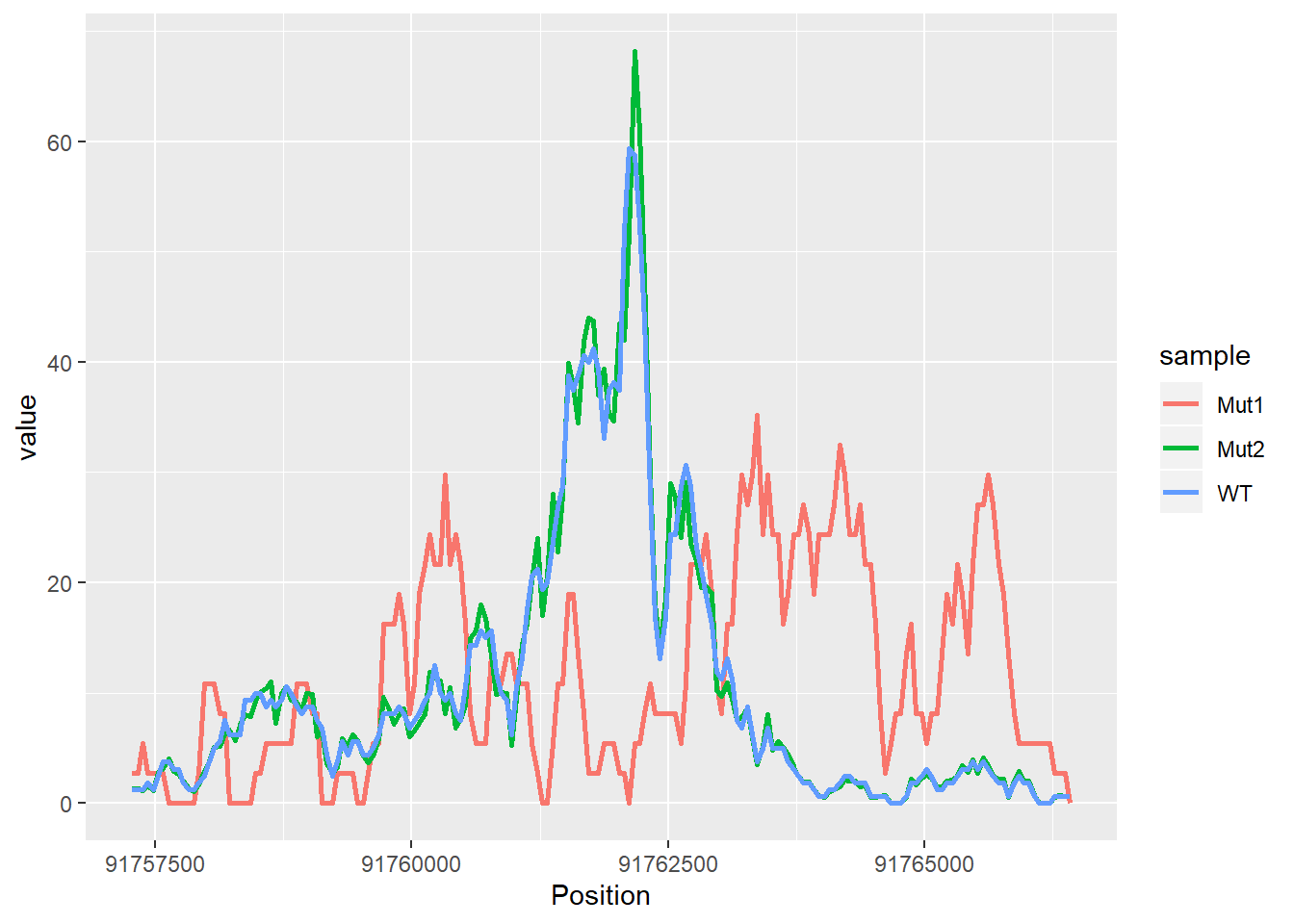## Genomes

Finally we’re going to look at the genome size vs number of chromosomes and colour it by domain in our genomes data.

``read_csv("genomes.csv") -> genomes``
``````## Parsed with column specification:
## cols(
##   Organism = col_character(),
##   Groups = col_character(),
##   Size = col_double(),
##   Chromosomes = col_double(),
##   Organelles = col_double(),
##   Plasmids = col_double(),
##   Assemblies = col_double()
## )``````
``head(genomes)``

To get at the `Domain` we’ll need to split apart the Groups field.

``````genomes %>%
separate(col=Groups, into=c("Domain","Kingdom","Class"), sep=";") -> genomes

``````genomes %>%